# regular polygon

Regular Polygon

A polygon for which all sides are congruent and all angles are congruent.

 Regular Polygon Formulasn = number of sidess = length of a sider = apothem (radius of inscribed circle)   R = radius of circumcircle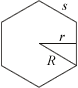Sum of interior angles = (n – 2)·180°Interior angle =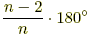Area = (½)nsr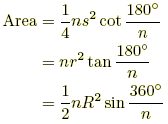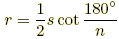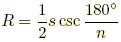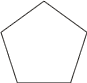Regular Pentagon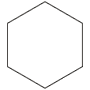Regular Hexagon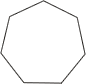Regular Heptagon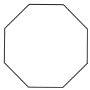Regular Octagon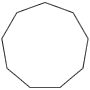Regular Nonagon